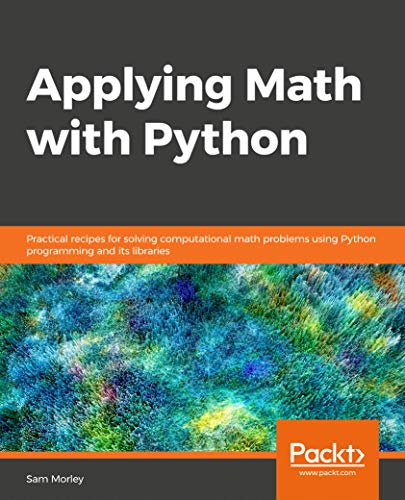# Applying Math with Python: Practical recipes for solving computational math problems using Python programming and its libraries

• Length: 327 pages
• Edition: 1
• Publisher:
• Publication Date: 2020-08-11
• ISBN-10: 1838989757
• ISBN-13: 9781838989750
Description

Discover easy-to-follow solutions and techniques to help you to implement applied mathematical concepts such as probability, calculus, and equations using Python’s numeric and scientific libraries

#### Key Features

• Compute complex mathematical problems using programming logic with the help of step-by-step recipes
• Learn how to utilize Python’s libraries for computation, mathematical modeling, and statistics
• Discover simple yet effective techniques for solving mathematical equations and apply them in real-world statistics

#### Book Description

Python, one of the world’s most popular programming languages, has a number of powerful packages to help you tackle complex mathematical problems in a simple and efficient way. These core capabilities help programmers pave the way for building exciting applications in various domains such as machine learning and data science, using knowledge in the computational mathematics domain.

The book teaches you how to solve problems faced in a wide variety of mathematical fields such as calculus, probability, statistics and data science, graph theory, optimization, and geometry. You’ll start by developing core skills and learning about packages covered in Python’s scientific stack, including NumPy, SciPy, and Matplotlib. As you advance, you’ll get to grips with more advanced topics of calculus, probability, and networks (graph theory). After you gain a solid understanding of these topics, you’ll discover Python’s applications in data science and statistics, forecasting, geometry, and optimization. The final chapters will take you through a collection of miscellaneous problems including working with specific data formats and accelerating code.

By the end of this book, you’ll have an arsenal of practical coding solutions that can be used and modified to solve a wide range of practical problems in computational mathematics and data science.

#### What you will learn

• Get familiar with basic packages, tools, and libraries in Python for solving mathematical problems
• Explore various techniques that will help you to solve computational mathematical problems
• Understand the core concepts of applied mathematics and how you can apply them in computer science
• Discover how to choose the most suitable package, tool, or technique to solve a certain problem
• Implement basic mathematical plotting, change plot styles, and add labels to the plots using Matplotlib
• Get to grips with probability theory with the Bayesian inference and Markov Chain Monte Carlo (MCMC) methods

#### Who This Book Is For

This book is for professional programmers and students looking to solve mathematical problems computationally using Python. Advanced mathematics knowledge is not a requirement, but a basic knowledge of mathematics will help you to get the most out of this book. The book assumes familiarity with Python concepts of data structures.

Free sampleTry Audible »
Cloud Hosting by Vultr

Subscribe
Categories
Tags
Source Code